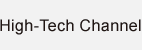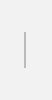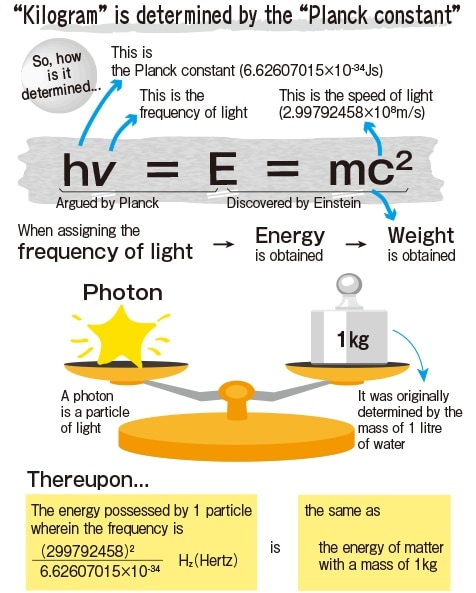### “Light” determines this unit of “weight”

Symbol: kg / Base data: mass. SI International System of Units
Kilogram is defined by taking the fixed numerical value of the Planck constant h to be 6.62607015 × 10-34Js.

The unit of mass is determined by the “Planck constant”

Matter with the same mass possess the same energy. This concept argues that mass is defined by the energy possessed by a single naturally-occurring photon. The Planck constant is the smallest unit for calculating energy possessed by that photon.Revised in 2021